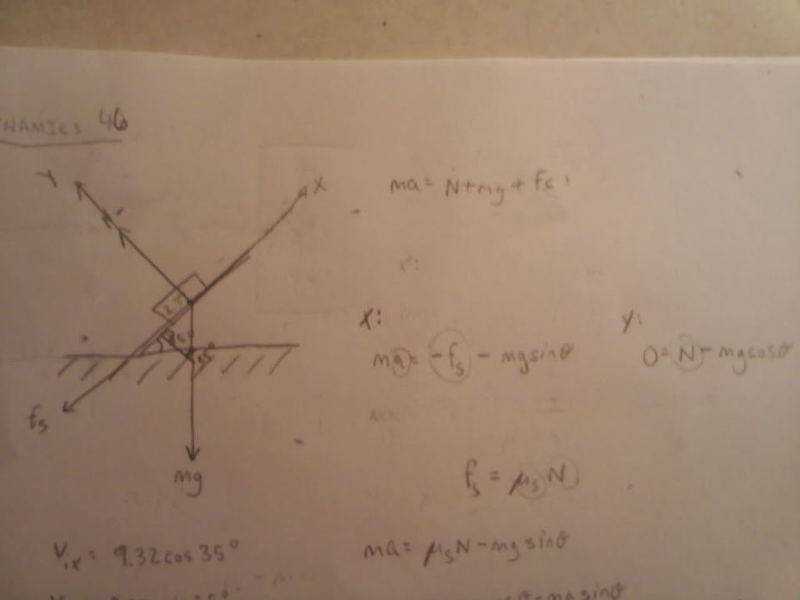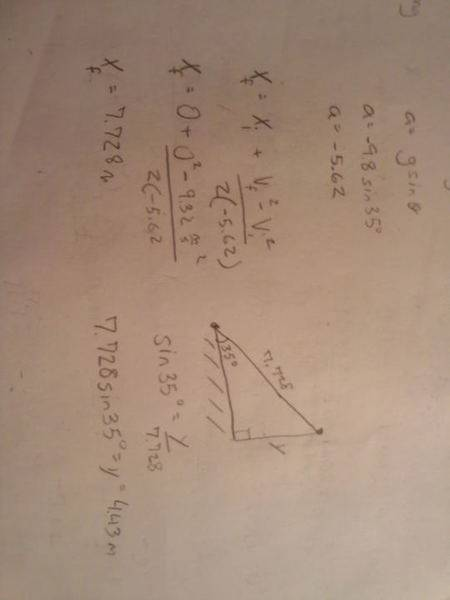# Block of wood pushed up a wooden ramp

## Homework Statement

A 2.37 kg wood block is launched up a wooden ramp that is inclined at a 35° angle. The block’s initial speed is 9.32 m/s. What vertical height does the block reach above its starting point?

## Homework Equations

DYNAMICS

3. The Attempt at a Solutionno matter what I do i end up with a=g(ukcos(theata)+sin(theata)) is this right how am i setting it up wrong?

Doc Al
Mentor
no matter what I do i end up with a=g(ukcos(theata)+sin(theata)) is this right how am i setting it up wrong?
Assuming there is friction (your first post doesn't mention any), that's the correct expression for the acceleration of the block along the incline. Was the problem statement in your first post complete?

I believe so. I took fs=us*n....and replaced fs with us*n. Is that wrong?

or should i assume there is no friction?

Doc Al
Mentor
I believe so. I took fs=us*n....and replaced fs with us*n. Is that wrong?
Your initial problem statement doesn't mention friction or give a value for μ. What makes you think there's friction involved here?

I think friction should be involved because its wood on wood, and when wood rubs against wood there is friction. Am i just thinking to much about this problem? If there is no friction then my acceleration would be just be gsin(theata). Correct?

Doc Al
Mentor
I think friction should be involved because its wood on wood, and when wood rubs against wood there is friction.
Most likely, but this is a physics problem so often things are ignored. You can only tell by context. For example, are you given a chart of coefficients of friction to use?
I suspect so.
If there is no friction then my acceleration would be just be gsin(theata). Correct?
Correct.

We weren't given a table there is one in the book but there is no wood on wood coefficient given. Thank you Doc Al, I am going to take the appoarch without friction and see how it goes and post back on here with what I come up with thank you.

Ok so i got the acceleration, which is negative because it slow down so can i just use Vf=Vi+(a*delta t) to find the time and then just use the kneimatics equation yf=yi+ (Viy*deltat)-(.5*g*delta t^2)? I tried that and it was wrong. Do i subsitute the gravity with the accleration i solved for?

Doc Al
Mentor
Ok so i got the acceleration, which is negative because it slow down so can i just use Vf=Vi+(a*delta t) to find the time and then just use the kneimatics equation yf=yi+ (Viy*deltat)-(.5*g*delta t^2)? I tried that and it was wrong. Do i subsitute the gravity with the accleration i solved for?
Of course! Use the acceleration you figured out.

Be sure to convert your answer into the form they ask for: The vertical height, not the distance along the ramp.

You can also use a different kinematic equation that combines the two you are using into one step. Save a little work.

well isnt that why I am using Yf and Yi in the equation that will give me how high it went?

Doc Al
Mentor
well isnt that why I am using Yf and Yi in the equation that will give me how high it went?
No. The block moves along the ramp, you found its acceleration parallel to the ramp, and that equation will find the distance traveled along the ramp. But you can easily use that answer--with a bit of trig--to find the change in height.

ahhhhh. Making since now!

but wait.....wouldnt the equation i use to find the length it travels up the slope be.....
Df=Di+(Vi*delta t)-(.5*a*delta t^2). The Vi is the Intial velocity not with respect to the y axis? and then the a is the calculated a?

Doc Al
Mentor
but wait.....wouldnt the equation i use to find the length it travels up the slope be.....
Df=Di+(Vi*delta t)-(.5*a*delta t^2).
You could certainly use that if you knew (or figure out) the time. (You could also you a different kinematic formula; one that relates, a, v, and d together.)
The Vi is the Intial velocity not with respect to the y axis? and then the a is the calculated a?
Right. The initial velocity you were given is parallel to the ramp, and the a needed is the one you calculated.

Ok this is all my work but it still said its wrong......Doc Al
Mentor
Ok this is all my work but it still said its wrong......
Looks OK to me. If this is one of those online systems, they can be picky about significant figures.

The only other thing I can suggest is to do it over including friction. But unless they give you the coefficient, I don't see how you can do that.

yeah that was the initial problem. and wood to wood coefficient can be between .25-.60 so i dont see how i could even pick one. Your the man Doc Al! I would be completely lost with out u. Even when I dont get rid of any significant figures til the end i still get 4.430 so... I am just going to email my professor and tell him I did all the work right but it didn't work.

There is a second part to this problem that I am going to try using my numbers and see if I can get it right.........

What speed does it have when it slides back down to its starting point?

Doc Al
Mentor
There is a second part to this problem that I am going to try using my numbers and see if I can get it right.........

What speed does it have when it slides back down to its starting point?
That second part is trivial if there's no friction, so I suspect that your professor does intend you to include friction. (Which makes sense, else why specify wood on wood?) Perhaps you can email him for the μ he wants you to use.

This part isn't working either when i know all of the work is right....If I use the distance it traveled up the ramp which was 7.728 m. mhhm its ok I emailed him

yeah I emailed him. I sent him a picture of my work and asked if we were suppose to use friction and that if we are suppose to use it what coefficient does he want us to use. There is no other way that this can be solved can there?

Doc Al
Mentor
There is no other way that this can be solved can there?
Not that I can see.

Ok, I believe you haha. i appreciate everything you helpped me with! I understand where all the numbers come from and how to change them to what i need! I guess it didn't help that we are missing a bit of information! lol